# Calculate magnetic field strength vs distance

#### xchcui

Joined May 12, 2014
257
Hi.

I know there is a very simple formula to calculate the power density of RF radiation at a certain distance from the source by knowing the transmitted power source. Which is:G/4Πr2 (where 4Πr2 is the surface area of a sphere and G is the antenna gain).
Is there any simple formula to calculate the elf magnetic field strength at a certain distance from the source? For example:if i measured an 45 milligauss magnetic field(ELF:50-60Hz)when the gauss meter is close attached to the measured object,how can i calculate the magnetic field strength at a distance of 10cm,20cm,1m etc.?
Thanks.

#### Danko

Joined Nov 22, 2017
1,772
Is there any simple formula to calculate the elf magnetic field strength at a certain distance from the source?
See picture below and check link: https://www.researchgate.net/post/How-does-the-magnetic-field-strength-varies-with-distance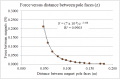Magnet calculator: https://magnetfabrik.de/en/knowledge/magnet-calculator
---------------------------------------------------------------------------------------------------------------------------
If someone could take my example(on my first post)with the 45 milligauss measurement(which was measured with gauss-meter that was attached at an electric device)and show me how he make the calculation to find the magnetic field strength at 20cm,70cm etc.from the device(in uT or mG units),it will be more than great.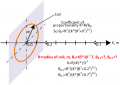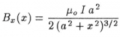Information in picture is corrected.

ALSO SEE !!!!!
https://archive.thepocketlab.com/educators/lesson/magnetic-field-axis-current-loop-0
AND THIS IS WHAT YOU REALLY NEED - Axial and Off-axis magnetic field of current loop - CALCULATOR
---------------------------------------------------------------------------
For better accuracy of measurements make coil with radius about 0.3...0,5 m (10...20 turns).
Do first measurement exactly in geometric center of coil.
-------------------------------------------------------------------------------------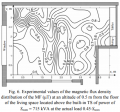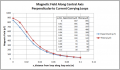See PDF...

#### Attachments

• 2 MB Views: 2
Last edited:
•cmartinez

#### xchcui

Joined May 12, 2014
257
If someone could take my example(on my first post)with the 45 milligauss measurement(which was measured with gauss-meter that was attached at an electric device)and show me how he make the calculation to find the magnetic field strength at 20cm,70cm etc.from the device(in uT or mG units),it will be more than great.

#### Tesla23

Joined May 10, 2009
537
Is there any simple formula to calculate the elf magnetic field strength at a certain distance from the source? For example:if i measured an 45 milligauss magnetic field(ELF:50-60Hz)when the gauss meter is close attached to the measured object,how can i calculate the magnetic field strength at a distance of 10cm,20cm,1m etc.?
Thanks.
No, and you can't, unless you know more about the source and it's orientation.

Electromagnetics would be much easier if you could predict the field everywhere from one simple measurement. In your case, the way the field drops off with distance depends on the source, and you can see this by looking at the near and far fields of canonical sources; line source, dipole, quadrupole etc..

#### Delta Prime

Joined Nov 15, 2019
1,311
Hello thereElectromagnetism is the branch of physics, which studies the forces that occur between electrically charged particles. An electromagnetic (EM) field is a physical field produced by moving electrically charged objects. It affects the behavior of charged objects in the vicinity of the field. The electromagnetic field can be viewed as a combination of an electric field and a magnetic field.
The magnetic flux density is also called "B field" or "magnetic induction".simple formulas do exist for less complex symmetrical geometries, enabling you to calculate the B field on a symmetry axis in north-south pole direction. Simple for you may be difficult for others it's all very subjective but doable good luck to you!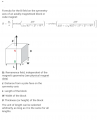#### xchcui

Joined May 12, 2014
257
Thanks for all the responses the links and the formula.
I have noticed that all the answers is related to magnets
included the formula,while i don't know what to do with that
in my specific case.
I didn't measure a magnet,i just attached EMF meter that
has gauss-meter option to an electric device(like transformer)
,took a reading(while the meter is attached to the transformer)and
checked how does the magnetic field strength drops vs distance.Now,
i am trying to find a way to calculate the magnetic-field strength of the transformer vs distance with a fimple formula in order to compare and check if the calculation is matching to the gauss-meter reading.

#### Delta Prime

Joined Nov 15, 2019
1,311
Hello there againi am trying to find a way to calculate the magnetic-field strength of the transformer vs distance with a fimple formula in order to compare and check if the calculation is matching to the gauss-meter reading.
Your enthusiasm is refreshing but now it's time for brash tactics.
Experimental research is a study that you have decided to embark upon.
It is my area of expertise and I offer you the following advice. Experimental research strictly adheres to a scientific research design. It includes a hypothesis, a variable that can be manipulated by the researcher, and variables that can be measured, calculated and compared. Most importantly,
experimental research is completed in a controlled environment. The researcher collects data and
results will either support or reject the hypothesis. This method of research is referred to a
hypothesis testing or a deductive research method. After
completing an experimental research study, a correlation between a specific aspect of an entity and
the variable being studied is either supported or rejected. Go get-em tiger!#### xchcui

Joined May 12, 2014
257
View attachment 233012
Danko,i have some question about that formula.
1)Is the letter K Coulomb constant?,which is-
~9·10^9 at units:N·m^2/C^2
2)What are the units for the R?
3)Should the unit for the K be changed in order that in my calculation
i will get the B result in mG?
4)If i take a measurement of the magnetic field strength
from an electric device with the gauss-meter,do i need to dismantle
the device in order to take the measurement,while the gauss-meter is attached to the device's coil in order to get accurate calculation result,
since the previous measurement of 45mG was taken,while the gauss-meter was attached to the device itself(some cm away from the coil)and not attached to the coil.Thanks.
Hello there againYour enthusiasm is refreshing but now it's time for brash tactics.
Experimental research is a study that you have decided to embark upon.
It is my area of expertise and I offer you the following advice. Experimental research strictly adheres to a scientific research design. It includes a hypothesis, a variable that can be manipulated by the researcher, and variables that can be measured, calculated and compared. Most importantly,
experimental research is completed in a controlled environment. The researcher collects data and
results will either support or reject the hypothesis. This method of research is referred to a
hypothesis testing or a deductive research method. After
completing an experimental research study, a correlation between a specific aspect of an entity and
the variable being studied is either supported or rejected. Go get-em tiger!procedure.I wasn't meant really to make a serious experimental research
and i am,apparently,not going to make any Sensational discoveries,
i am just checking my EMF meter reading(magnetic field strength/ELF) compare to theoretical formula,not a really big-deal.
But yes it still an Experimental.Thanks.

#### Janis59

Joined Aug 21, 2017
1,783
You stand in the bit of trap. There are zillions of formulas for DC where limiting factor is mju relative of air. There are enough formulas for RF where any source of RF energy is at the same order impedance as Free Space corresponding 376 Ohms. But ELF is a very special case where it is a question do the 376 ohms are still in the force and as well the air gap or different magnetic cores still both simultaneously may be working. Thus, if no core, let use a Friis formula but with caution. If interest is field around core, use a DC magnet core equation and both effective magnetic path lengthes but factually walk around with a measurer and validate do the figures compass. Sad to tell that widespread masurer TM195 is not good suited for ELF, but it newest competitor the GQ-390 is. At least for 50 Hz mains it works just brilliant. May tell the 1000 measurment sessions in few words - around ordinary home small power cable the zone at which World accredited Human health normative is overstepped is like inch to 4 inch distance. If the wire is going under the matress not beyound the bedsheet, all is OK. About high voltage lines it is bit longer tale. Depending on voltage and load the safe distance to build the residential house is from the 50 meters aside (20 kV) up to 200 meters aside (500 000 Volt). The health normatives are asessed for case of low frequencies ar ICNIRP-2010 (apply this as querry to Google).
PS And, if want to make a science around it, there are two brilliant magnetic modelling softwares along with damn expensive ANSYS Gaussian module, namely multiple hacks having Comsol and free Open GPL licence having LISA v7.

Thanks again for you all